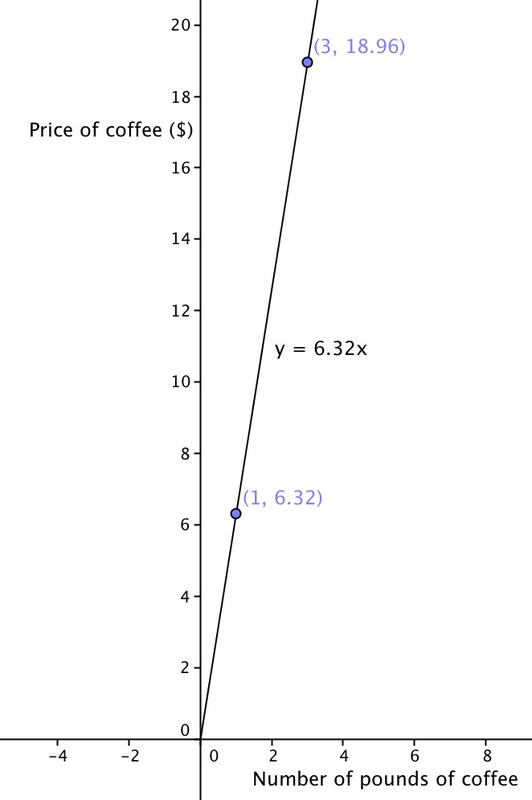Coffee costs \$18.96 for 3 pounds. 1. What is the cost for one pound of coffee? 2. At this store, the price for a pound of coffee is the same no matter how many pounds you buy. Let$x$be the number of pounds of coffee and$y$be the total cost of$x$pounds. Draw a graph of the relationship between the number of pounds of coffee and the total cost. 3. Where can you see the cost per pound of coffee in the graph? What is it? ## IM Commentary The purpose of this task is for students to find a unit rate in a context where two quantities are in a proportional relationship and to draw the graph of that proportional relationship. This is a task where it would be appropriate for students to use technology such as a graphing calculator or GeoGebra, making it a good candidate for students to engage in MP5, Use appropriate tools strategically. A variant of this problem is appropriate for 8th grade; see 8.EE.5 Coffee by the Pound. ## Solution 1. You can find the cost for one pound of coffee by dividing the total cost by 3. Coffee costs \$6.32 per pound.
3.4. The cost of one pound, \$6.32, may be seen on the graph in two ways: • As the point (1, 6.32) • As the slope of the line: \$6.32 per pound.